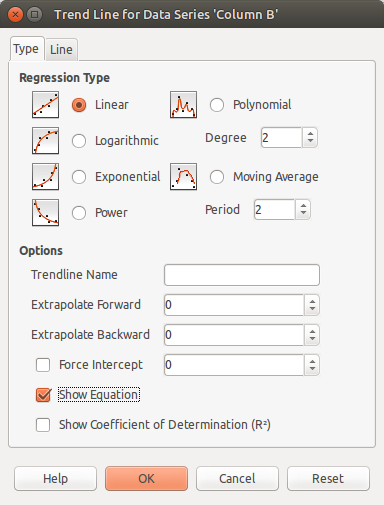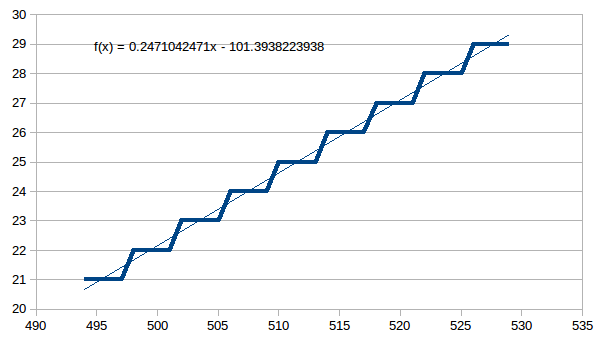## Converting ADC digital value into degrees Celsius

23 posts / 0 new
Author
Message

Hello !

I'm making a thermometer and I need to find an efficient way of converting the ADC digital value to degrees Celsius.

That's what I've done for now.

```switch (ADC){
case 496: temp = 21; break;
case 497: temp = 21; break;
case 498: temp = 21; break;
case 499: temp = 21; break;
case 500: temp = 22; break;
case 501: temp = 22; break;
case 502: temp = 22; break;
case 503: temp = 22; break;
case 504: temp = 23; break;
case 505: temp = 23; break;
case 506: temp = 23; break;
case 507: temp = 23; break;

}```

The actual code goes from 20 degrees to 30 and the list is already quite lengthy. What if I decide to make a temperature range from -40 to 40 degrees ? Is there a formula or something to make the conversation simpler and quicker ?

This topic has a solution.
Last Edited: Mon. Nov 21, 2016 - 02:41 AM
Total votes: 0

Plot your readings versus temperature on a graph. If it's a straight line you should be able to get the slope and crossing point for a y=mx + c function. If it's none linear you probably want to break out Excel and do some curve fitting to get the coefficients for some none linear function.

Total votes: 0

What are you using for a temperature sensor? There might be a library you can use and make your life easy.

Jim

I would rather attempt something great and fail, than attempt nothing and succeed - Fortune Cookie

"The critical shortage here is not stuff, but time." - Johan Ekdahl

"If you want a career with a known path - become an undertaker. Dead people don't sue!" - Kartman

"Why is there a "Highway to Hell" and only a "Stairway to Heaven"? A prediction of the expected traffic load?"  - Lee "theusch"

Speak sweetly. It makes your words easier to digest when at a later date you have to eat them ;-)  - Source Unknown

Please Read: Code-of-Conduct

Atmel Studio6.2/AS7, DipTrace, Quartus, MPLAB, RSLogix user

Total votes: 0

I'm using KTY81 110. And I made a graph.Is there a formula for this graph which will tell the temperature for the given ADC value ?

This reply has been marked as the solution.
Total votes: 1

Subtract 494 and divide the remainder by 4; then add 20.

Neil

Last Edited: Sun. Nov 20, 2016 - 05:32 PM
Total votes: 0

Oh my god, how did you come up with this ? I've been twisting my head like all day and did not make anything that would work. Thank you !

Total votes: 1

And to make it cleaner start at 0

subtract 414 and div with 4.

The 414 id 494-20/(1/4)  ;slope  = 1/4

Total votes: 0

How did you come up with that ? Is there some rule for this kind of slopes ?

Total votes: 0

Did you really go all the way through school with no one ever teaching you that a straight line was y=mx+c?

Total votes: 0

To be honest, I don't remember that from school, maybe I skipped that day when they were teaching that.

Total votes: 0

Well the modern student may well use some Regression and curve fitting software like Matlab or perhaps a spreadsheet rather than getting out the maths textbooks. Now your graph was almost certainly produced in Excel or similar so you were very nearly there. All you had to do was right click on the plot line and choose "Insert Trend Line". Here's Libreoffice doing that:You were lucky that KTY81 is highly linear and Neil Barnes could calculate a good fit in his head. A more non-linear sensor would provide more of a mental challenge which is where software like this is a massive boon.

PS: You should be able to identify how Neil's (divide 4) maps to the linear regression equation.

PPS: That plot line wasn't created by experimentation was it ? Did you actually use an equation to generate it ?

Total votes: 0

Yeah I've got the calculations to their place. I made the graph on some random website and draw it buy putting the coordinates of the points. With KTY81 110 things are quite linear from 20 to 30 but get a little bet more curvy from there on.

Total votes: 0

I thought there may be a short-cut but I just pulled up the datasheet. They don't give an equation; only a temperature / resistance table at (mostly) 10°C steps. So drawing a graph and regression analysis is the way to go.

Total votes: 0

I think the easiest way would be drawing polynomial regression in Excel or a similar program.

Total votes: 0

This thread intrigued me - I wondered how you could get LibreOffice to do such a curve fit so I went googling and found this Youtube video...

https://www.youtube.com/watch?v=...

It was worth the price of entry just to see how this guy moans about what a real engineer would really want (and not want)! :-)

Total votes: 0

An engineer would never use so many superlatives...

 "Experience is what enables you to recognise a mistake the second time you make it." "Good judgement comes from experience.  Experience comes from bad judgement." "Wisdom is always wont to arrive late, and to be a little approximate on first possession." "When you hear hoofbeats, think horses, not unicorns." "Fast.  Cheap.  Good.  Pick two." "We see a lot of arses on handlebars around here." - [J Ekdahl]Total votes: 0

His teachings are strong.

"No scientist or engineer would ever consider drawing this graph"

- A guy from that video.

Total votes: 0

How did you come up with that ? Is there some rule for this kind of slopes ?

clawson

Did you really go all the way through school with no one ever teaching you that a straight line was y=mx+c?

To be honest, I don't remember that from school, maybe I skipped that day when they were teaching that.

less than a day later........

I think the easiest way would be drawing polynomial regression in Excel or a similar program.

Someone is a very fast learner.

David

Total votes: 0

N.Winterbottom wrote:

<...>

You were lucky that KTY81 is highly linear and Neil Barnes could calculate a good fit in his head. A more non-linear sensor would provide more of a mental challenge which is where software like this is a massive boon.

PS: You should be able to identify how Neil's (divide 4) maps to the linear regression equation.

PPS: That plot line wasn't created by experimentation was it ? Did you actually use an equation to generate it ?

N Winterbottom, you live around the corner from me!

My approach was a little more ad-hoc, based on simple examination of the signal rather than actually plotting a line - though of course the process is mathematically the same.

1. assumption: this is coming from some sort of digital sensor; these are generally quite linear in the area of interest
2. assumption: the output is actually not drawn correctly (the software has joined the dots); inspection shows that there are four values for each degree <--- here's the divide by four
3. the first value mapped to 20C was 494 - so subtract that and you'll get a value of 0 for 20C, 4 for 21C, 8 for 22C etc.
4. do the divide by four so you get a value above 20C
5. and as the last stage add 20 to get an actual temperature value.

Neil

Total votes: 0

LightningWalrus wrote:
Oh my god, how did you come up with this ? I've been twisting my head like all day and did not make anything that would work.

Ummm--Algebra 101?  Or is it Trig or Geometry?  Anyway, I'd assume if one was going to do work such as this, there would be familiarity with y=mx+b.

N.Winterbottom wrote:
PPS: That plot line wasn't created by experimentation was it ? Did you actually use an equation to generate it ?

It certainly looks "regular", but the slanty slopes are confusing, as well as the same exact degree result all the time, spanning several ADC counts.

You can put lipstick on a pig, but it is still a pig.

I've never met a pig I didn't like, as long as you have some salt and pepper.

Total votes: 0

LightningWalrus wrote:

How did you come up with that ? Is there some rule for this kind of slopes ?

He got that from the table you posted... When the reading increases by 4, the temperature increases by one, so slope (Δx/Δy) = 1/4. The slope is m in y=mx+b. You can then calculate b.

```switch (ADC){
case 496: temp = 21; break;		//21.0
case 497: temp = 21; break;		//21.25
case 498: temp = 21; break;		//21.5
case 499: temp = 21; break;             //21.75
case 500: temp = 22; break;		//22.0
case 501: temp = 22; break;	        //and so on...
case 502: temp = 22; break;
case 503: temp = 22; break;
case 504: temp = 23; break;
case 505: temp = 23; break;
case 506: temp = 23; break;
case 507: temp = 23; break;

}```

Total votes: 0

theusch wrote:
It certainly looks "regular", but the slanty slopes are confusing

Well the guy in the video did say:

"No scientist or engineer would ever consider drawing this graph"

Perhaps he was right after all.Total votes: 0

barnacle wrote:

Subtract 494 and divide the remainder by 4; then add 20.

Neil

Wow thank you! easy peasy

mikkesskoteruthyrning

Last Edited: Thu. Apr 27, 2017 - 02:10 PM